Design measurement circuit for given codes
Weilei Zeng
Department of Physics & Astronomy, University of California, Riverside, California 92521, USA
(Dated: January 28, 2020)
There are many equivant transformation for elementary quantum gates, which introduces diﬀerent
circuits for the same computation or measurements. Those circuits have the same function, but vary
in depth, qubit connectivity, overhead of ancilla qubits, and etc. It is still unkown how to optimize
a circuit for optimal performance for given tasks and devices. This work discusses some handy
modiﬁcations to reduce the circuit size, including utilizing additional qubits and modifying order of
gates.
CONTENTS
List of Figures 1
Introduction 1
Basics of Quantum Circuit 2
Optimization for Shor’s 9-qubit Code with additional
ancilla qubits 2
Optimizing circuit for a small Quantum
Convolutional code, by changing gate order 3
References 4
LIST OF FIGURES
1 CNOT
01
= CNOT (|q
0
i, |q
1
i) . . . . . . . . . . . 2
2 measure Y
0
= Z
0
X
0
, input state α(|0i +
i |1i) + β(|0i i |1i) . . . . . . . . . . . . . . . . . . . . 2
3 measure Z
0
Z
1
, input state α |00i + β |11i . 2
4 measure X
0
X
1
X
2
X
3
X
4
X
5
, input state
α(|0i + |1i)
6
+ β(|0i |1i)
6
. . . . . . . . . . . 3
5 Circuit equivalent to Fig.4 . . . . . . . . . . . . . . . 3
6 Circuit equivalent to Fig.4, time steps de-
crease by half . . . . . . . . . . . . . . . . . . . . . . . . . . 3
7 Circuit for Shor’s code, optimized to
have the minimal time steps. Input state
α(|000i + |111i)(|000i + |111i)(|000i +
|111i) + β(|000i |111i)(|000i
|111i)(|000i |111i) . . . . . . . . . . . . . . . . . . . . 3
8 Circuit to measure (111|1ωω), 7 time steps 4
9 Circuit equivalent to Fig.8, 5 time steps . . . 4
10 G =
1 1 1 1 ω ω
1 1 1 1 ω ω
. . . . . . . . . . 4
11 G =
1 1 1 1 ω ω
ω ω ω ω ω 1
1 1 1 1 ω ω
ω ω ω ω ω 1
. . . . . . . . 4
12 G =
1 1 1 1 ω ω
ω ω ω ω ω 1
1 1 1 1 ω ω
ω ω ω ω ω 1
1 1 1 1 ω ω
ω ω ω ω ω 1
5
13 G =
1 ω ω
ω ω 1
1 1 1 1 ω ω
ω ω ω ω ω 1
1 1 1 1 ω ω
ω ω ω ω ω 1
1 1 1 1 ω ω
ω ω ω ω ω 1
1 1 1
ω ω ω
6
14 conv4v2 . . . . . . . . . . . . . . . . . . . . . . . . . . . . . . . 7
INTRODUCTION
The elementary gates  in quantum circuits have
many equivalent relations. For example, X
2
= H
2
= I,
HZH = X, and a CNOT with four Hadamard gates is
just a CNOT in inverse order, i.e. H
i
H
j
CNOT
ij
H
i
H
j
=
CNOT
ji
. Those relations allow a circuit for a given task
to have many equivalent forms, which vary in depth,
qubit connectivity, overhead of ancilla qubits, and etc.
As a result, those circuit may perform diﬀerently in ex-
cution time, error propagation and fault tolerance. It is
more complicated, when one has to apply it on a quan-
tum device, which adds constraints on qubit connectivity
and availabity of gates.
Many quantum platforms are working on such opti-
mization [citation needed]. They allow as many gates on
the user interface, but then has to translate it into a ver-
sion with limited gates and qubit connectivity allowed
by the device. These tranlation are done in the back-
end. Another solution was presented by Lingling Lao
during QIP 2020 [citation needed]. They develop a pro-
gram which can quantify the constraints of a quantum
device and translate a general quantum circuit to one
that satisfy those constraint.
In practice, most quantum people working on algo-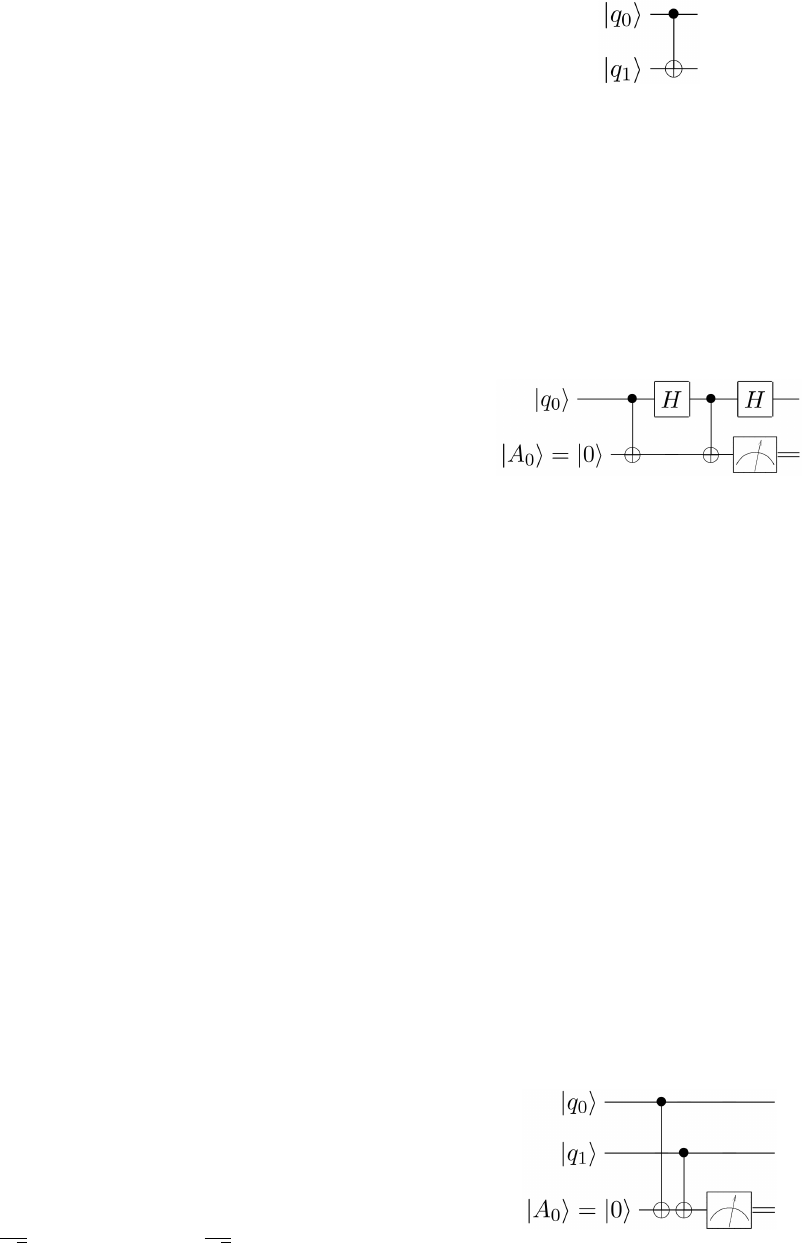2
rithm or error correction, are not master of optimizing
circuit, or it is less eﬃcient to spending time on it. Peo-
ple usually assume some simple circuit model and start
building circuit. For example, one may assume one can
reset a qubit to |0i any time. But resetting in a quantum
device actually takes signiﬁcant long time to calibrate,
and is usually not allowed in devices for a single task.
In this work, we are unable to give an optimal way
of designing a circuit. But we do introduce some handy
techniques to reduce the size of a circuit. Here the size is
the product of number of qubit and circuit depth, which
is positively related to the error probabilities in the cir-
cuit.
Our design is based on a circuit error model. We de-
vide the circuit into diﬀerent time steps/frames. After
each step, we introduce a random X or Z error with
p
X
= p
Z
= p. This model include single gate error,
two-qubit gate error, and also idle error. It also include
measurment errors, which corresponds to the qubit er-
rors right before measurement gates. One can add an-
other layer of measurement error to the classical bits of
measurement syndrome.
The optimization is based on two source. First, one
can utilize the equivalent relations to reduce the number
of gates used. Second, for a stabilizer code, one can the-
oretically do all stabilizer measurement in parallel. This
can be used to make the circuit more compact and reduce
the depth of circuit.
BASICS OF QUANTUM CIRCUIT
A quantum circuit consists of a sequence of elementary
gates. For simulation of quantum error correction, if it
only contain Cliﬀord gates, the circuit can be simulated
classically. Here we introduce some basics relations of the
Cliﬀord gates {X, Y, Z, H, S, CNOT } (note that T gate
is not Cliﬀord gate).
Pauli gates, Hadamard gate and Phase gate
X
2
= Y
2
= Z
2
= H
2
= S
2
= I
H and S can change the basis (ignore the overall phase)
HZH = X, HXH = Z (1)
SXS = Y, SY S = X, SZS = Z (2)
Our measurement gate always use the basis of eigen
states of Z,
spin up Z |0i = |0i, spin down Z |1i = |1i
H |0i =
1
2
(|0i |1i), H |1i =
1
2
(|0i + |1i)
CNOT
01
= CNOT (|q
0
i, |q
1
i) is shown in Fig.1
FIG. 1. CNOT
01
= CNOT (|q
0
i , |q
1
i)
In an earlier version of our note, we didn’t use S gate
to change the basis while meausuring Y , but we use the
following circuit with two CN OT gates.
Lemma 1. To measure Y , one can either use S gate to
change the basis, or measure both X and Z on the same
qubit, as shown in Fig.2
FIG. 2. measure Y
0
= Z
0
X
0
, input state α(|0i+i |1i)+β(|0i
i |1i)
Usually, one can use only one ancilla qubit to measure
one generator. But some times multiple ancilla qubits
can reduce time steps or ﬁt cirtain qubit connectivity.
The following lemma explains how to split the measure-
Lemma 2. To measure a generator g = g
0
g
1
, one can
use two ancilla qubits |a
0
i and |a
1
i to measure g
0
and g
1
separately. Then apply CNOT (|a
0
i, |a
1
i) and measure
|a
1
i
For each code and error model, there should be a bal-
ance between the cost of additional ancilla qubits and the
reduction in time steps. See example in Fig.5 and Fig.6
in the next Section for a case study.
OPTIMIZATION FOR SHOR’S 9-QUBIT CODE
Shor’s code has two types of stabilzier generators,
weight-2 Z operators and weight-6 X operators.
The circuit for measuring Z
0
Z
1
is given by Fig.3
FIG. 3. measure Z
0
Z
1
, input state α |00i + β |11i
The circuit for measuring X
0
X
1
X
2
X
3
X
4
X
5
is given by
Fig.4 By reversing the CNOT gate, it can be transformed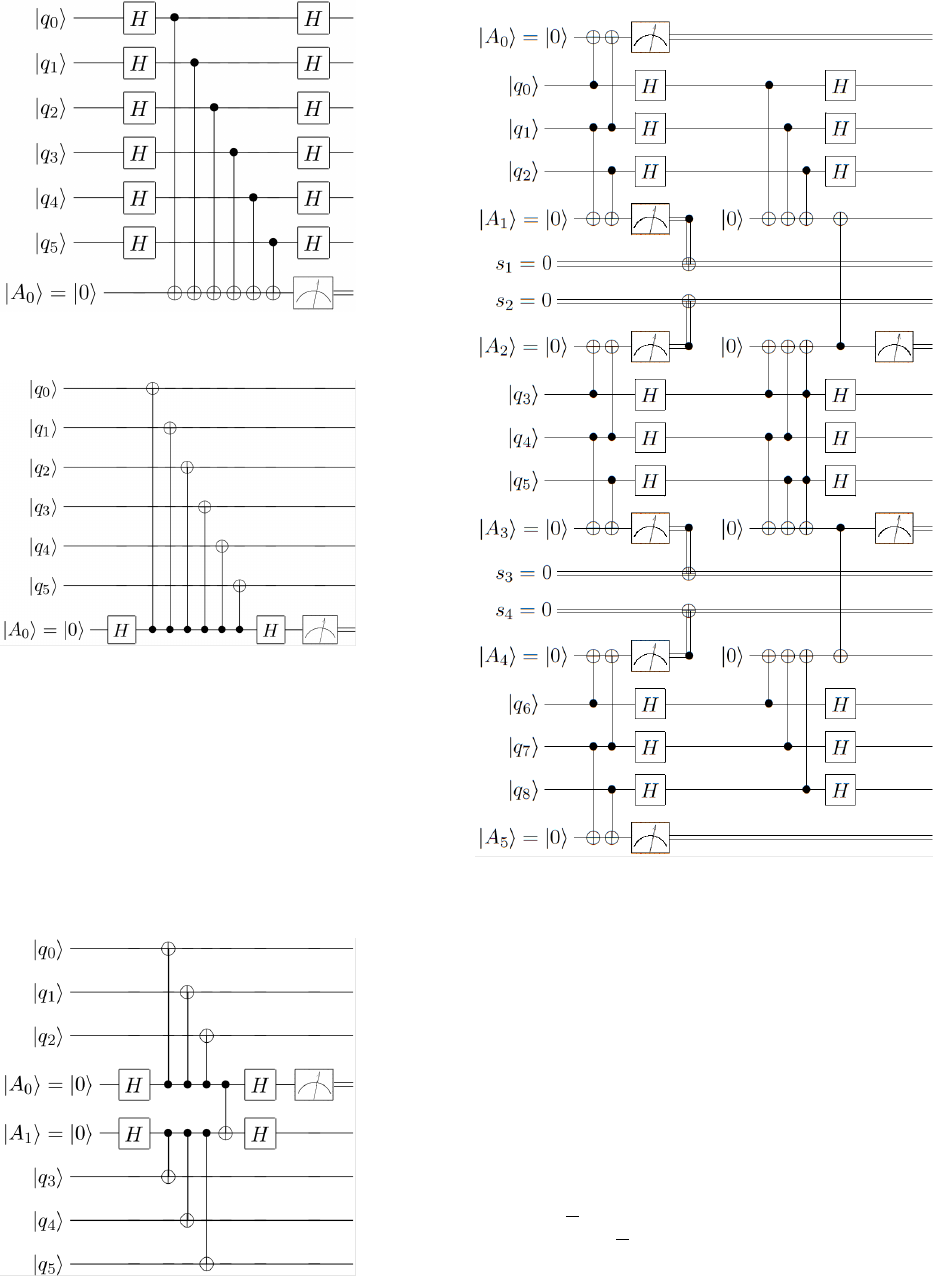3
FIG. 4. measure X
0
X
1
X
2
X
3
X
4
X
5
, input state α(|0i +
|1i)
6
+ β(|0i |1i)
6
FIG. 5. Circuit equivalent to Fig.4
into an equivalent one, as shown by Fig.5, which require
less H gates.
To decrease the time frames, one can add more ancilla
qubits and apply Lemma.2. This yields another equiva-
lent circuit in Fig.6
Combine these techniques together, Fig.7 gives the op-
FIG. 6. Circuit equivalent to Fig.4, time steps decrease by
half
FIG. 7. Circuit for Shor’s code, optimized to have the minimal
time steps. Input state α(|000i + |111i)(|000i + |111i)(|000i +
|111i) + β(|000i |111i)(|000i |111i)(|000i |111i)
timized circuit for Shor’s codes. The ancilla qubits get
doubled, but time steps are reduced by about 1/3.
In the next section, we use another example to show
how to use parallel measurement to reduce the circuit
size.
OPTIMIZING CIRCUIT FOR A SMALL
QUANTUM CONVOLUTIONAL CODE, BY
CHANGING GATE ORDER
In this section, we discuss the circuit for various codes
based on a GF(4) linear convolutional code with genera-
tors (111|1ωω).We ﬁrst show the circuit to measure one
generator (111|1ωω) in Fig.8. It is equivalent to Fig.9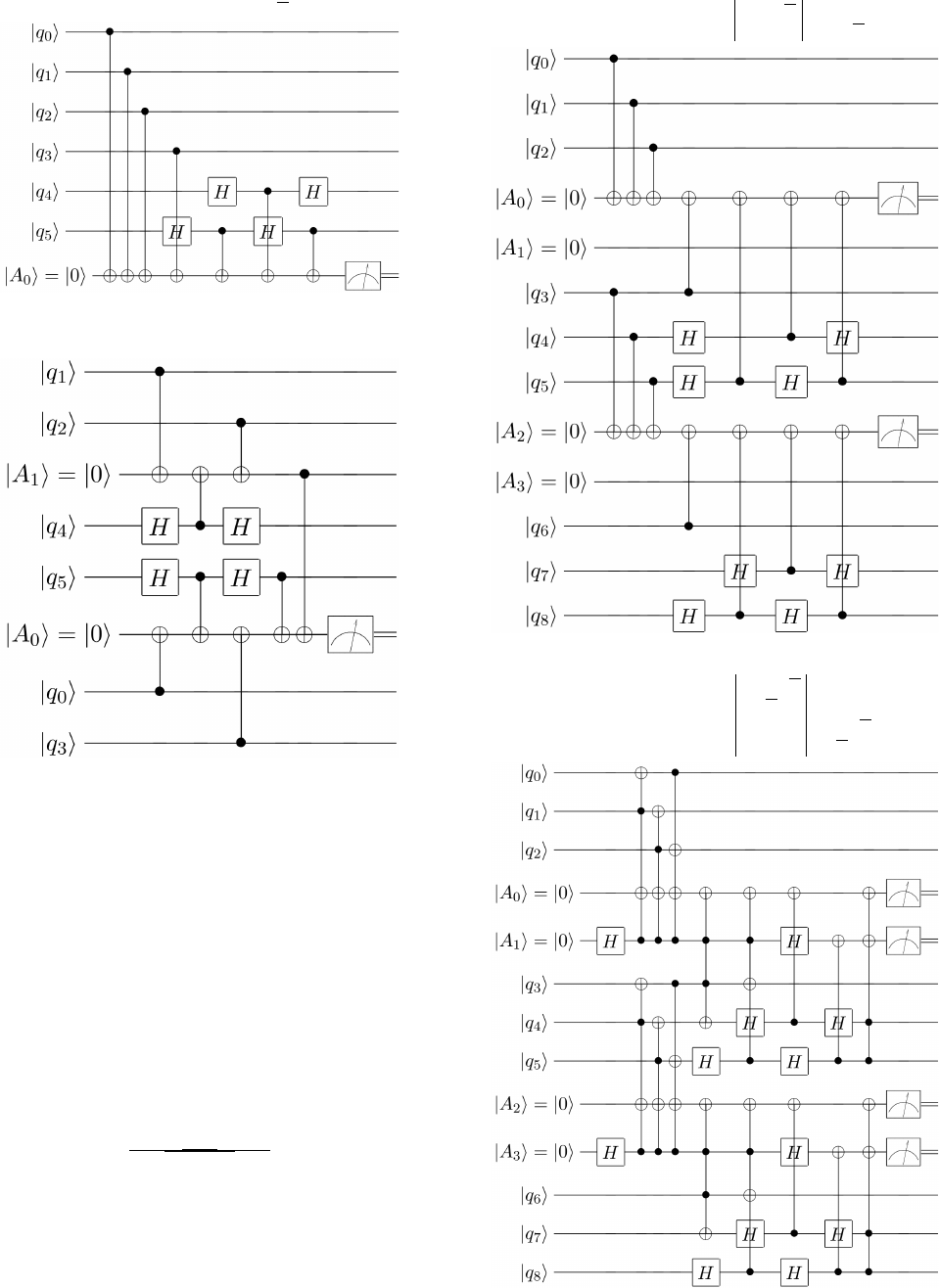4
FIG. 8. Circuit to measure (111|1ωω), 7 time steps
FIG. 9. Circuit equivalent to Fig.8, 5 time steps
Let’s compare Fig.8 with Fig.9. Fig.8 has 7 times step
and an ancilla qubit for each three qubits. It has circuit
size (3 + 1) ×7 = 28 unit. Fig.9 has 5 times step and two
ancilla qubits for each three qubits. Its size is (3+2)×5 =
25 unit. There is no much reduction in this case. Later
on when we change the code to larger size, I will just use
Fig.8.
Now deﬁne several bigger codes in Fig.10, Fig.11 and
Fig.13, with generator matriices given in the captions.
What these examples show is that, one can change the
order of gates appropriately to reduce the number of idle
qubits (qubit waiting for other gates to ﬁnish). This can
reduce the time steps of the circuit.
weilei.zeng@email.ucr.edu
 Adriano Barenco, Charles H Bennett, Richard Cleve,
David P DiVincenzo, Norman Margolus, Peter Shor, Ty-
cho Sleator, John A Smolin, and Harald Weinfurter, “Ele-
mentary gates for quantum computation,” Physical review
FIG. 10. G =
1 1 1 1 ω ω
1 1 1 1 ω ω
FIG. 11. G =
1 1 1 1 ω ω
ω ω ω ω ω 1
1 1 1 1 ω ω
ω ω ω ω ω 1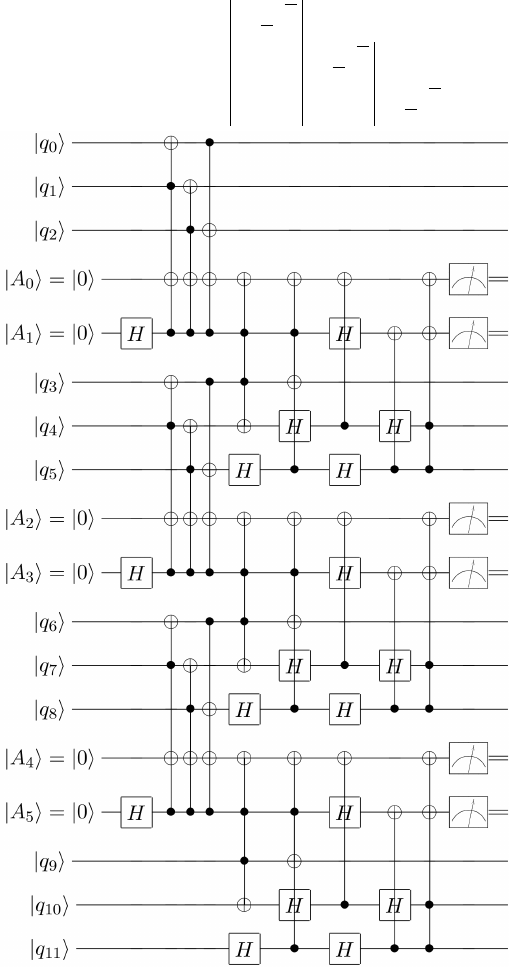5
FIG. 12. G =
1 1 1 1 ω ω
ω ω ω ω ω 1
1 1 1 1 ω ω
ω ω ω ω ω 1
1 1 1 1 ω ω
ω ω ω ω ω 1
A 52, 3457 (1995).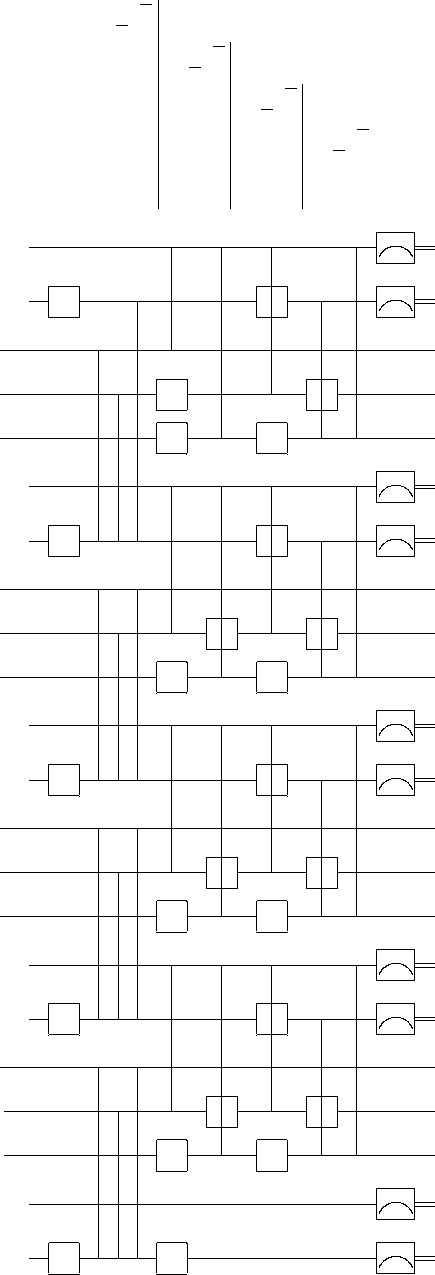6
FIG. 13. G =
1 ω ω
ω ω 1
1 1 1 1 ω ω
ω ω ω ω ω 1
1 1 1 1 ω ω
ω ω ω ω ω 1
1 1 1 1 ω ω
ω ω ω ω ω 1
1 1 1
ω ω ω
|q
0
i
|A
0
i = |0i
LL
|A
1
i = |0i
H
H
LL
|q
0
i
|q
1
i
H
H
|q
2
i
H
H
|A
2
i = |0i
LL
|A
3
i = |0i
H
H
LL
|q
3
i
|q
4
i
H
H
|q
5
i
H
H
|A
4
i = |0i
LL
|A
5
i = |0i
H
H
LL
|q
6
i
|q
7
i
H
H
|q
8
i
H
H
|A
6
i = |0i
LL
|A
7
i = |0i
H
H
LL
|q
9
i
|q
10
i
H
H
|q
11
i
H
H
|A
8
i = |0i
LL
|A
9
i = |0i
H
H
LL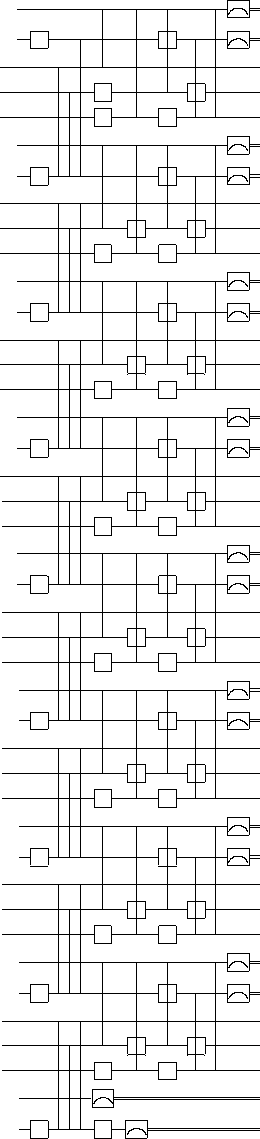7
FIG. 14. conv4v2
|q
0
i
|A
0
i = |0i
LL
|A
1
i = |0i
H
H
LL
|q
0
i
|q
1
i
H
H
|q
2
i
H
H
|A
2
i = |0i
LL
|A
3
i = |0i
H
H
LL
|q
3
i
|q
4
i
H
H
|q
5
i
H
H
|A
4
i = |0i
LL
|A
5
i = |0i
H
H
LL
|q
6
i
|q
7
i
H
H
|q
8
i
H
H
|A
6
i = |0i
LL
|A
7
i = |0i
H
H
LL
|q
9
i
|q
10
i
H
H
|q
11
i
H
H
|A
8
i = |0i
LL
|A
9
i = |0i
H
H
LL
|q
12
i
|q
13
i
H
H
|q
14
i
H
H
|A
10
i = |0i
LL
|A
11
i = |0i
H
H
LL
|q
15
i
|q
16
i
H
H
|q
17
i
H
H
|A
12
i = |0i
LL
|A
13
i = |0i
H
H
LL
|q
18
i
|q
19
i
H
H
|q
20
i
H
H
|A
14
i = |0i
LL
|A
15
i = |0i
H
H
LL
|q
21
i
|q
22
i
H
H
|q
23
i
H
H
|A
16
i = |0i
LL
|A
17
i = |0i
H
H
LL